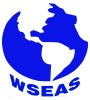Plenary Lecture

Solution of Eddy Current Testing Problems for Media with Axisymmetric Flaws of Finite SizeProfessor Andrei Kolyshkin
Department of Engineering Mathematics
Riga Technical University
LATVIA
E-mail: andrejs.koliskins@rbs.lv

Abstract: In the present talk we consider two approaches for the solution of eddy current problems for media with axisymmetric flaws. The first approach is based on a perturbation method where a small parameter represents the difference between the electrical conductivites of a flaw and a surrounding conducting medium. Approximate change in impedance of a coil due to the flaw in the form of a circular cylinder of finite size is found in terms of a double integral containing Bessel functions. In addition, the applicability of the layer approximation to the solution of axisymmetric problems with flaws is also discussed.
The second approach uses the TREE method in order to construct semi-analytical solutions of the problem. The main idea of the TREE („Truncated Eigenfunction Expansions”) method is that the vector potential is assumed to be exactly zero at a sufficiently large radial distance from the coil. The corresponding boundary value problem for the vector potential in each region of interest can be solved by the method of separation of variables. The method is not truly analytical since two aspects of the procedure require the use of numerical methods. First, a set of complex eigenvalues has to be determined numerically. Second, a system of linear algebaric equations for the expansion coefficeints has to be solved numerically as well. Different types of problems including axisymmetric flaws are considered. Results of numerical simulations are presented. Calculated results are compared with available experimental data.

Brief Biography of the Speaker: Andrei Kolyshkin received his undergraduate degree in Applied Mathematics in 1976 at the Riga Technical University. In 1981 he received a Ph.D in differential equations and mathematical physics at the University of St. Petersburg (Russia). Andrei Kolyshkin is currently a full professor at the Department of Engineering Mathematics at the Riga Technical University. His current research interests include investigation of stability problems in fluid mechanics with applications to open- channel flows, transient flows in hydraulic systems and mathematical models for eddy current testing. He is the co-author of three monographs published by Academic Press and CRM. Andrei Kolyshkin has participated in more than 40 international conferences and has published more than 70 papers in refereed journals since 1980. As a visiting professor and visiting researcher he spent a few years at the University of Ottawa and Hong Kong University of Science and Technology.

## Bulletin Board

Currently:

The Conference Program is online.

The Conference Guide is online.